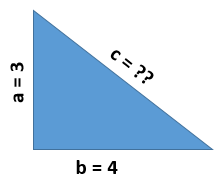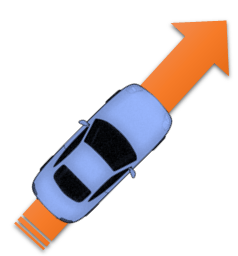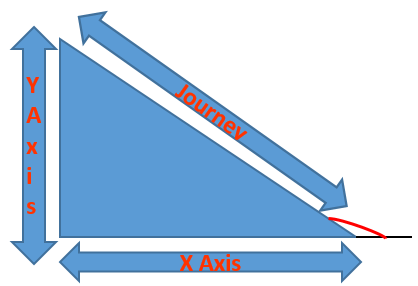# Maths In Games Pythagorus and TrigonometryThis is the 4th post in my Maths In Games series, and this is covering the topic that started this whole series.

The day I was working on coding a pinball game and I had to apply Pytagorean Theorem and I had a flash back to my school days where I had to sit through Maths classes wondering “when am I ever going to use this in the real world!?!”.A good place to start is probably to remind ourselves what Pythagorus was saying.

In the right-angle triangle below we can see that the length of a and b below is 3 and 4 respectively, but how do we find out the length of c?

Pythagorus tells us that the sum of the square of a and b gives us the square of c.

So in the case of our example below (3*3) + (4*4) = c squared, which gives us 25.  The square root of 25 is 5, which is the length of c.How does that help us when creating games?

## Maths In Games: Pythagorean Theorem and Reach

If our character is waving a bat around with a length of 10, you might want to know if his bat will reach an enemy.

If your character and enemy are side by side with the same Y position then it is easy to calculate the difference between your character’s X position and the enemy’s X position, if this is 10 or less then your character can reach and hit the enemy.

If they are not on the same level  though it is not as straight forward.

Our blue character below is our hero, and the orange character is an enemy.

Can our hero’s bat hit the enemy?  Obviously from this image it won’t reach, but if they were closer together how can you work out if the bat will reach or not?The blue arrow above shows the distance between the two characters along the X axis.

The green arrow above shows the distance on the Y axis and the orange arrow shows the real distance between them, if the bat is longer than this distance then it will reach the enemy.

Let’s assume our character, c, is at position 5 on the X and Y axis and our enemy, e, is at 17 on the X axis and 10 on the Y axis.  Will our characters bat, which is 10 long, manage to hit the enemy from here?Let’s get the differences between our character and enemy on the X and Y axis.

• X axis : 17 – 5 = 12
• Y axis : 10 – 5 = 5

12 and 5 can be thought of the lengths of 2 sides of our triangle.  If we square these and add them together we get the distance between our character and enemey.

(12 * 12) + (5 * 5) = 144 + 25 = 169 which is 13 squared.

The distance between our character and enemy is 13, our character’s bat will not reach the enemy.

## Maths In Games : Pythagorean Theorem and Speed

If we were working on a car game, and using a similar example to the triangle above, and our car was travelling at a speed of 12 on the X axis and 5 on the Y axis, then our car is travelling diagonally, the true speed of our car (using the Pythagorean Theorem above) would be 13.

This true speed could be displayed on screen, or in a chase game you might have police chase your car once your true speed went over 10 for example.

## Maths In games : Tennis and Changing Angles

Can you imagine playing a game of tennis where the ball just bounces back and forth from left to right.  It would be a really easy game to get a high score in, but it would also be an incredibly boring game!

For games like tennis, pong, pinball, etc. you want the angle that the ‘ball’ is sent back at to change depending on what part of the racket/paddle/etc. the ball hits and also what part of the ball hits the racket/paddle/etc.

For this series of posts I won’t get into the coding example too much because it can vary so much between languages.

For this section I’ll use the old game Breakout as an example.In this game, you controlled a paddle at the bottom of the screen and had to bounce a ball to break the colored blocks at the top of the screen.

This would have been an extremely boring game if the ball just dropped down, hit your paddle and bounced straight back up.

Using maths the angle the ball bounces back up at can be changed based on the part of the paddle the ball hits.

The red/orange arrows are the movement of the ball, it hits the paddle slightly off-center, so bounces back up at a small angle.

The green arrows represent another movement of the ball, the ball hits the paddle far from center so bounces back at a bigger angle.After playing the game enough times you might see that there are ways that you can control the movement of the ball better.  This can be made more realistic by considering the movement of the paddle as it hits the paddle, the angle the ball is travelling at before it hits the paddle, etc.

You could keep adding to your physics equation to make the ball movement more realistic.

## Maths In Games : Driving More Trigonometry

Trigonometry deals with triangles and doesn’t stop just at Pythagorus, there are also other riveting (note sarcastic tone) topics like sine, cosine, tangents, radians and degrees.

One of the few mnemonics I could remember from my school days was SOHCAHTOA, Sin=Opposite/Hypotenuse; Cosin=Adjacent/Hypotenuse and Tan=Opposite/Adjacent.

I could always remember the mnemonic but had no idea what it meant!

These are used in games where you know the angles and need to know the lengths of sides, or where you know the lengths and need to calculate the angles.

Think of a game that looks down on your character or vehicle (the original Grand Theft  Auto games were like this).

Your vehicle may start facing upwards and you might use the “up” arrow to accelerate.If we stuck with our previous movements  along the x and y axes, while playing the game your car turns left, the “up” arrow was what you used to accelerate initially would now be replaced by the “left” arrow.  But this isn’t the case when playing games like this.Or, if you car was rotated at a less straightforward angle, you would imagine that you would need the “up” and “right” arrows at the same time, but again this isn’t the case.As well as movement along the X and Y axes, in games like this we also include rotation in our movement instructions.

In the image below, our car has speed along the X and Y axis.  Pythagorus’s theorem gives us the hypotenuse to complete this triangle and also gives the line the car will travel along (until its movement changes).It is not enough to move the car along this line, it would look pretty strange if it faced up like above and moved along the journey line.

It would look better if the car rotated and traveled along the line, then it looks more convincing like a car turning and moving forward.

In the image below I have replaced the car with a line and a red arc.

The angle between the line and the triangle (under the red arc) is the angle used to calculate what the car needs to rotate by.

We know the opposite and adjacent, the tan calculation could give us the angle inside the triangle below, but we need to know the inverse angle (arctan) outside the triangle below.When we rotate the car, the “up” arrow will still be used to accelerate the car and not to just move the car along the Y axis.

How is this done?

If you think that our car can rotate 360 degrees, or right around a circle, split up this circle and pick a point on the circumference, from this you can start to see a triangle within the circle.

The way I think of this is if the car was rotated at the angle in the circle below, and you accelerated the car (using the “up” key) the car will move along the highlighted line in the circle below.This is how we could use the “up” key to mean accelerate the car, rather than just move the car up the Y axis.

As some of these concepts get more difficult, it is hard to explain generically how the code might look.

Instead I’ve decided to go through some basic game concepts using HTML5 and Canvas, for this you only need a basic word editor (I usually use Notepad) and an internet browser.

In the next posts I will run through creating small elements of games and then put some of them together, by recreating these yourself I hope they make more sense.

But for now, think back on some of your favorite classic games and see if you can figure out how maths played a part in them.

I suggest thinking of older or 2d games first because it can be easier to spot triangles and figure out the maths behind the game, more recent games that play in a 3d world have a third dimension thrown in.

As well as the X and Y axes, there is also a Z axis to contend with.## Canvas Examples

Since some parts of these posts have been difficult to explain while trying to use generic examples, the next few posts will show you how to create game elements yourself using just a text editor and browser.

This also gives you the chance to change certain elements (X and Y positions, etc.) and to see the effects yourself.